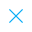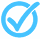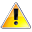Call Support +91-85588-96644

Keep me logged in
You can't leave Captcha Code empty
By submitting this form, you agree to the Terms & Privacy Policy.
ORTests given# Linear Equations in Two Variables - Test - 2 By TCY All tests

Questions

Time

Highest
score

Level

## ENGLISH

Language

Topics Covered:Linear Equations with One and Two VariablesSolving Pair of Linear Equations by Elimination MethodGraphical Method of finding the Solutions of a Pair of Linear EquationsMathematicsSolving a Pair of Linear EquationsAlgebraic Methods of Finding the Solutions for a Pair of Linear EquationsGraph of Equation ax + by + c = 0Pair of Linear Equations in Two VariablesSolution of System of Linear Equations in 2 VariablesSolutions of Linear Equations in 2 Variables